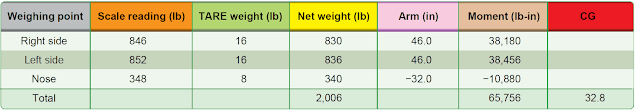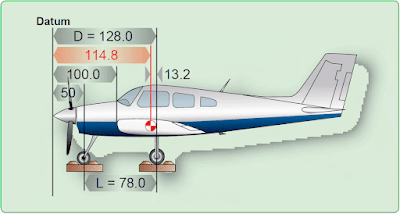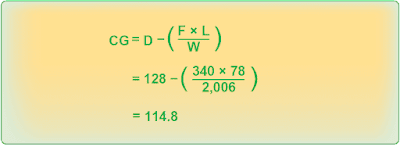# EWCG Formulas

A chart such as the one in Figure 1 helps the pilot visualize the weights, arms, and moments when solving an EWCG problem, but it is quicker to determine the EWCG by using formulas and an electronic calculator. The use of a calculator for solving these problems is described in Section 8, Use of Computers in Weight and Balance Computations.Figure 1. Locating the CG of an airplane relative to the datum

There are four possible conditions and their formulas that relate the location of CG to the datum. Notice that the formula for each condition first determines the moment of the nosewheel or tailwheel and then divides it by the total weight of the airplane. The arm thus determined is then added to or subtracted from the distance between the main wheels and the datum, distance D.

## Datum Forward of the Airplane—Nosewheel Landing Gear

The datum of the airplane in Figure 2 is 100 inches forward of the leading edge of the wing root or 128 inches forward of the main-wheel weighing points. This is distance (D). The weight of the nosewheel (F) is 340 pounds, and the distance between main wheels and nosewheel (L) is 78 inches. The total weight of the airplane (W) is 2,006 pounds. Determine the CG by using the formula in Figure 3.Figure 2. The datum is 100 inches forward of the wing root leading edgeFigure 3. Determining the CG with datum forward of an airplane with nosewheel landing gear

The CG is 114.8 inches aft of the datum. This is 13.2 inches forward of the main-wheel weighing points, which proves the location of the datum has no effect on the location of the CG if all measurements are made from the same location.

## Datum Aft of the Main Wheels—Nosewheel Landing Gear

The datum of some aircraft may be located aft of the main wheels. The airplane in this example is the same one just discussed, but the datum is at the intersection of the trailing edge of the wing with the fuselage. The distance (D) between the datum of the airplane in Figure 4 and the main-wheel weighing points is 75 inches, the weight of the nosewheel (F) is 340 pounds, and the distance between main wheels and nosewheel (L) is 78 inches. The total net weight of the airplane (W) is 2,006 pounds.Figure 4. The datum is aft of the main wheels at the wing trailing edgeFigure 5. Determining the CG with datum aft of the main wheels of an airplane with nosewheel landing gear

The location of the CG may be determined by using the formula in Figure 5.

The CG location is a negative value, which means it is 88.2 inches forward of the datum. This places it 13.2 inches forward of the main wheels, exactly the same location as when it was measured from other datum locations.

RELATED POSTS